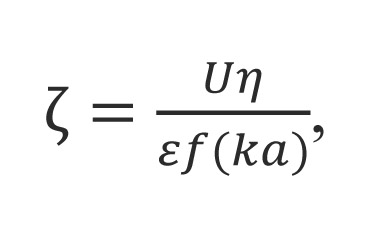Many nanoparticles or colloidal particles have a surface charge when they are suspension. When an electric field is applied, the particles move due to the interaction between the charged particle and applied field. The direction and velocity of the motion is a function of particle charge, the suspending medium, and the electric field strength. Particle velocity is then measured by observing the Doppler shift in the scattered light. The particle velocity is proportional to the electrical potential of the particle at the shear plane which is zeta potential. Thus, this optical measurement of the particle motion under an applied field can be used to the determine zeta potential.Particle motion under an applied electric field is known as electrophoresis. The method used by SZ100 is known as laser Doppler electrophoresis. Sample particles are suspended in a solvent af known refractibe index n, velocity η and dielectric constant ε. The sample is irradiated with laser light of wavelength λ. An electric field with strength E is applied. Due to the electric field, the particles are moving. Since the particles are moving, the scattered light at angle ϑ is measured and the particle velocity V is determined from the frequency shift. Mobility is then readily obtained as the radio of velocity to electric field strength V/E. Zeta potential is then found mobility using a model, the most common of which is the Smulochowski model.The following equation is used for the relationship between the calculated electrical mobility and zeta potential.where ζ – Zeta potential, U – Electrical mobility, E – Electric field strength, ε – Solvent dielectric constant, n – Solvent refraction index, η – Solvent viscosity, f(ka) – henry coefficient

Overviews and methods

Under construction

Equipment

Laser particle analyzer SZ100

Contacts

The Leading Researcher in optical systems Alexandr Shimko

Specialist in spectroscopy and granulometry Anastasia Povolotckaia

Specialist in optical systems Alexandra Mikhaylova